# Time Series analysis for Facebook message volume

2017-12-01

## Introduction to the data set

The data is the amount of Facebook messages a user (me) sent and received between 2011 and 2016. The data was exported from Facebook, converted from HTML to JSON and subsequently read into R. For more details on how this was done, please consult here.

The original data source contains 413,101 timestamps and messages between 2008 and 2016. The volume of messages is very low between 2008-2011, and highly atypical to the rest of the series, so it was not considered in the sample. An example is shown below:

In order to create a suitable format for time series analysis, the padr package was used in order to fill in missing observations with null values and aggregate all timestamps into weekly measurements. Message volume is defined as the quantity of messages sent and received by the user.

rawTs<- df %>%mutate(date = lubridate::with_tz(date,"CET"))%>% # change the time zone
thicken( interval = "month") %>% #creates a column with date_month
group_by(date_week) %>% # groups the date hour observations
summarise(amount = n()) %>% # counts the amount of messages sent or recieved
pad %>% # creates missing values for intervals where observations are missing
fill_by_value(amount)%>%   # fill missing values with 0
as_tbl_time(date_month) %>% # creating a new object class to ease time filtering
time_filter(2011 ~ 2016) # filter to 2011-2016

Now the data is in a suitable format to create time series objects.A train set was created for the first three years, with a test set created for the final year. Both were adjusted to account for calendar effects.

totalTs <- ts(data = rawTs[,2],
start = c(2011,1),# start
end = c(2016,3), # end with the last month omitted
frequency =12  #months in a year
)
end = c(2015,2))
test.ts <- window(totalTs.adj,start = c(2015,3),end = c(2016,3))

## Exploration of data

It is evident before any exploration that we are limited by the relativly short duration of the time series at the monthly level and the fact that the message volume starts at 0. The formet indicates that the ability to forecast seasonal patterns may be limited and the latter contradicts the stationarity assumption. It would be preferable to have several decades of measurements. However, neither the author nor Facebook were around several decades ago, so we will have to proceed taking these limitations into account.

In order to gain an understanding of the data set exploratory plots of the training set were made. The most informative subset of these are displayed below. First we begin with a time series plot.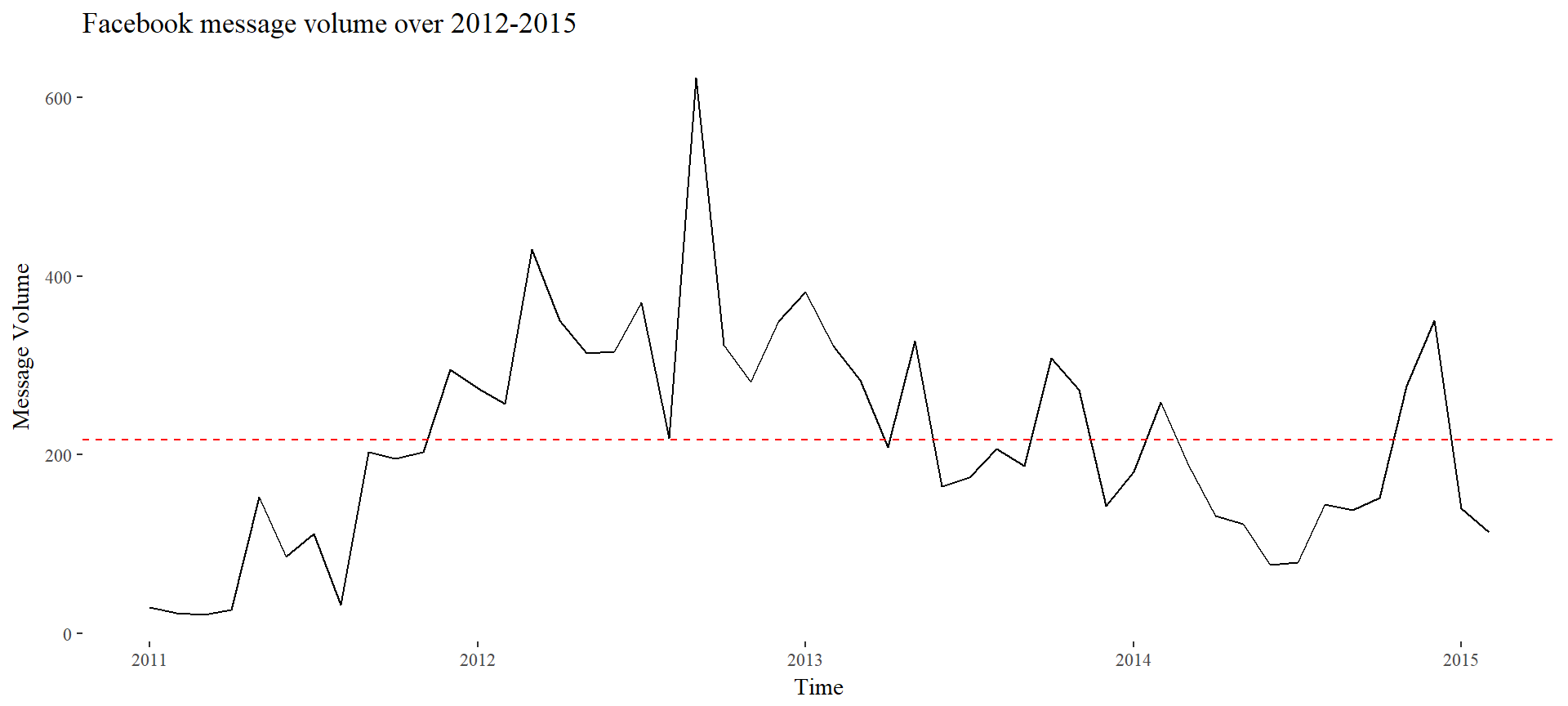Time series plot of the daily volume of message sent and recieved by the user. The dashed red line indicates the mean over the period.

The times series plot shows an upwards trend with a peak in 2012. There is a slight downwards trend, with a recovery towards the mean towards the end of the series. In addition, the variance does not seem to change significantly over time, although perhaps you could make the argument that there is less variance at the end and beginning of the series compared to the middle.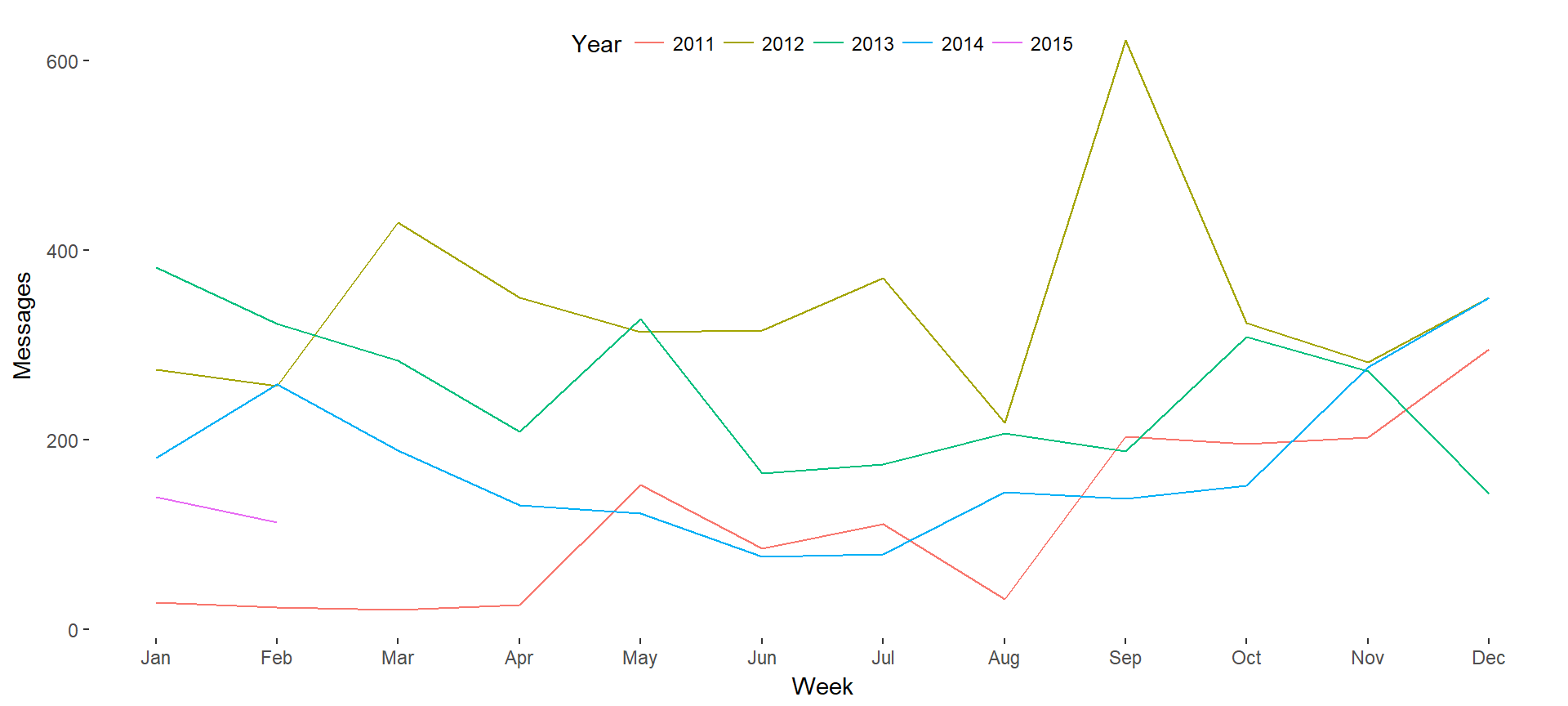Seasonal plot of the user’s message volume. There are no obvious trends.

In the plot above there are no obvious seasonal effects. There, for instance, dips around the last weeks of the year 2013 and 2012, but the exact opposite is observed in 2011 and 2014.

As an aside, the absense of seasonality is interesting because it depends entirely on the unit of measurement. If we take the same data but hourly instead of monthly, clear trends are visible with regards to the circadian rhythm.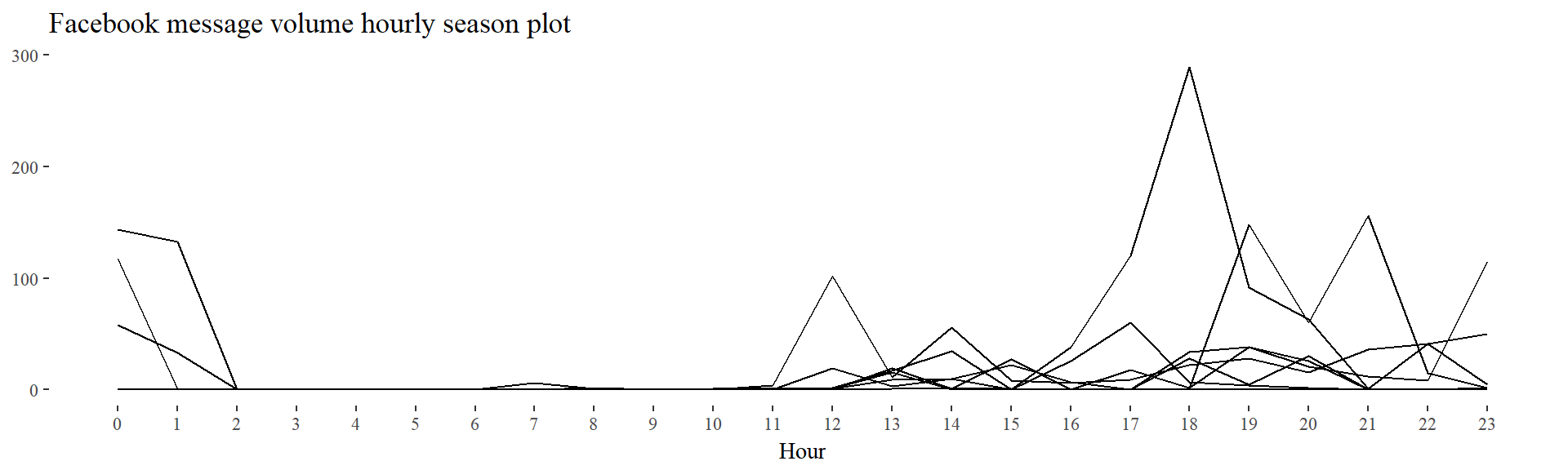An exploratory seasonplot of hourly Facebook message volume for the first week of 2012.

The inverted V-shaped trend is more visible in the month plot. This confirms the observation made that the volume of messages sent seems to increase and subsequently decrease.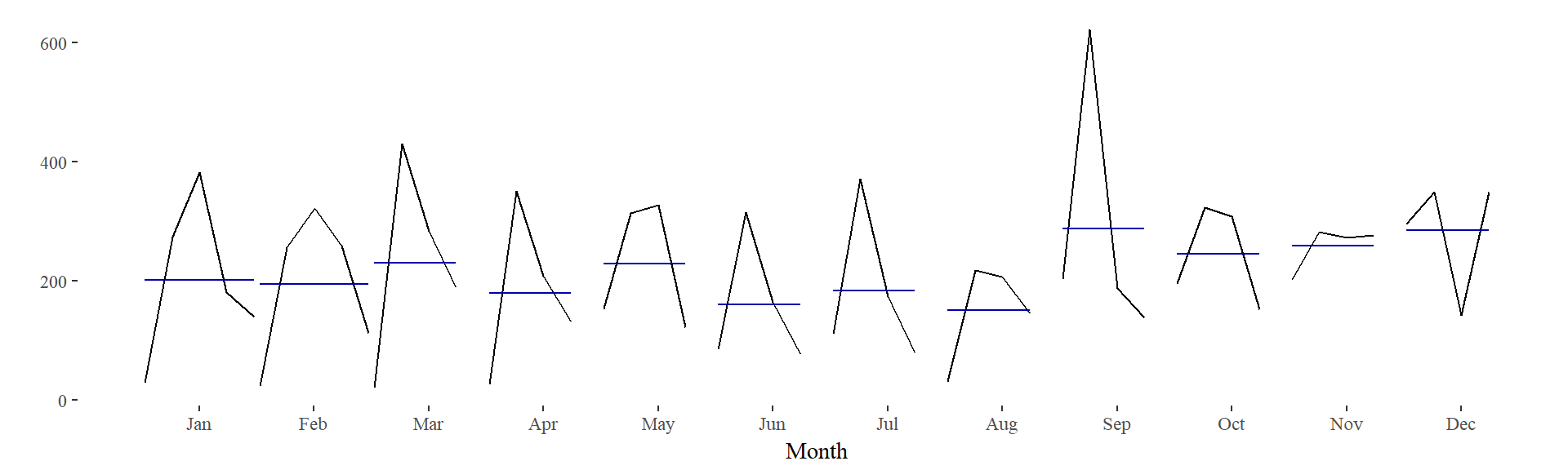A month plot of the series. Here the downwards trend following an upwards trend is most evident for most months.

## Exploring possible transformations

Based on the exploration of the data, whereby the series was roughly horizontal, with relatively constant variance and no predictable patterns in the long term, I decided to check whether the weak stationarity property holds. I did this using the sample ACF plot, the Augmented Dickey-Fuller Test as well as the KPSS Test for Level Stationarity.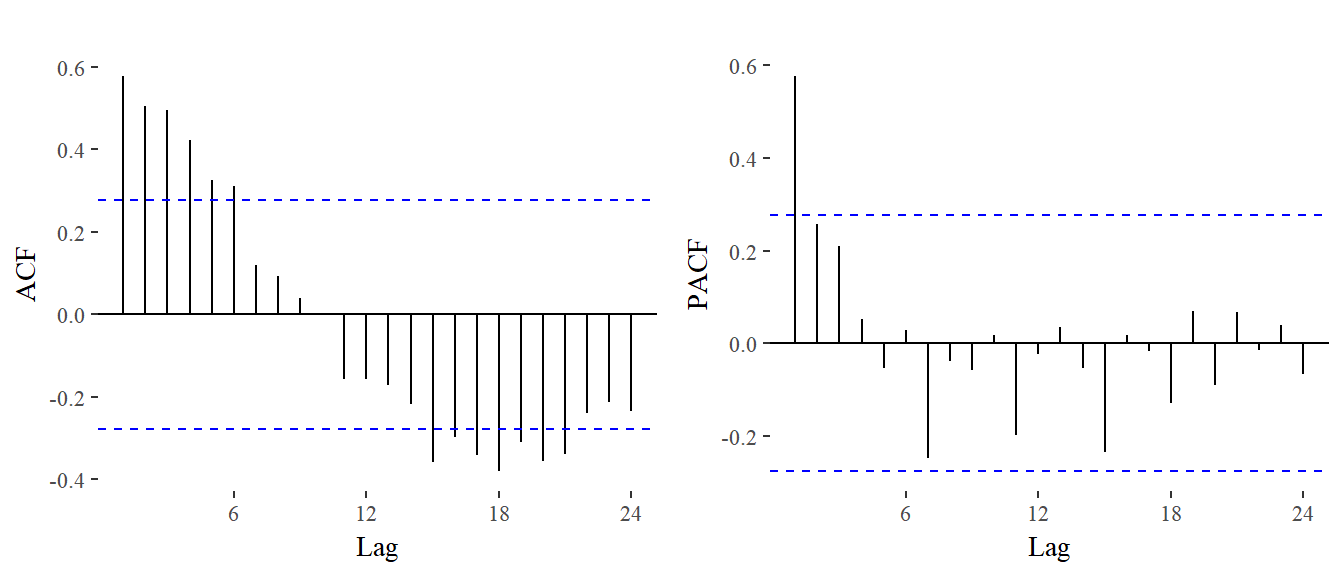Sample ACF and PACF plot of Facebook message volume.


Augmented Dickey-Fuller Test

data:  train.ts
Dickey-Fuller = -2.2507, Lag order = 3, p-value = 0.4737
alternative hypothesis: stationary
##
KPSS Test for Level Stationarity

data:  train.ts
KPSS Level = 0.42622, Truncation lag parameter = 1, p-value =
0.06585

The sample ACF plot does not indicate cleary stationarity and neither does the KPSS test, although the ADF test finds little evidence against non-stationarity. As these results are ambigous, differencing could be used to transform the data. Yet the nsdiffs() function returns 0 so no differencing was applied.

Another possible transformation would be a BoxCox transformation to stabilise the variance. Using the Guerrero method, the parameter for the transformation estimated was $$\lambda$$ = 0.931537. The transformed time series is plotted below.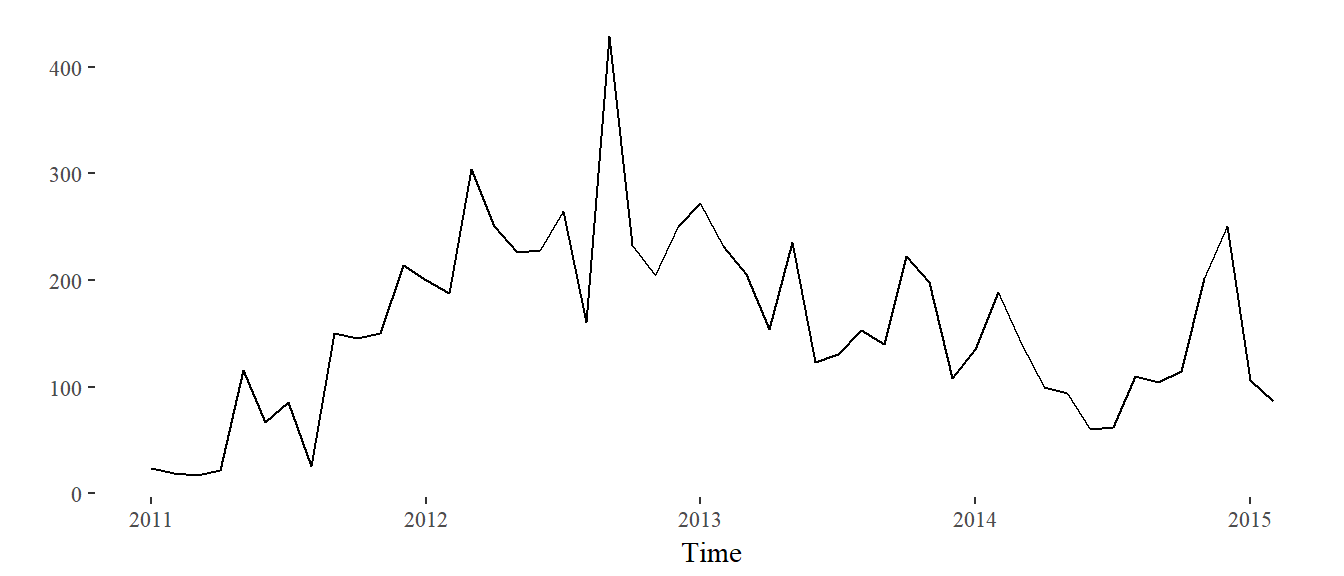Box-Cox transformed training test series.

I decided to not to apply this transformation as it does not change much and limits interpretability. Moreover, the auto.arima() and ets() functions evaluate whether to apply these transformations, so there was no need to apply them to the data beforehand.

## Modelling

### Choosing a benchmark model

Two possible benchmark models would be the naive model and the mean forecast. Unsurprisingly, both of the models have unacceptable residuals, with significant autocorrelations in the ACF chart, and the Ljung-Box test rejecting the null hypothesis that they are not correlated.

naiveModel <- naive(train.ts,h = 12)
meanModel <- meanf(train.ts,h = 12)

The graphical analyses of the residuals were omitted from the report, however both show substantial autocorrelation and the distribution of the residuals do not appear normal, as judged both visually and through the Ljung-Box test.

### Arima family

mArima1<- auto.arima(train.ts)
fa1 <- forecast(mArima1,h = 12)
checkresiduals(mArima1)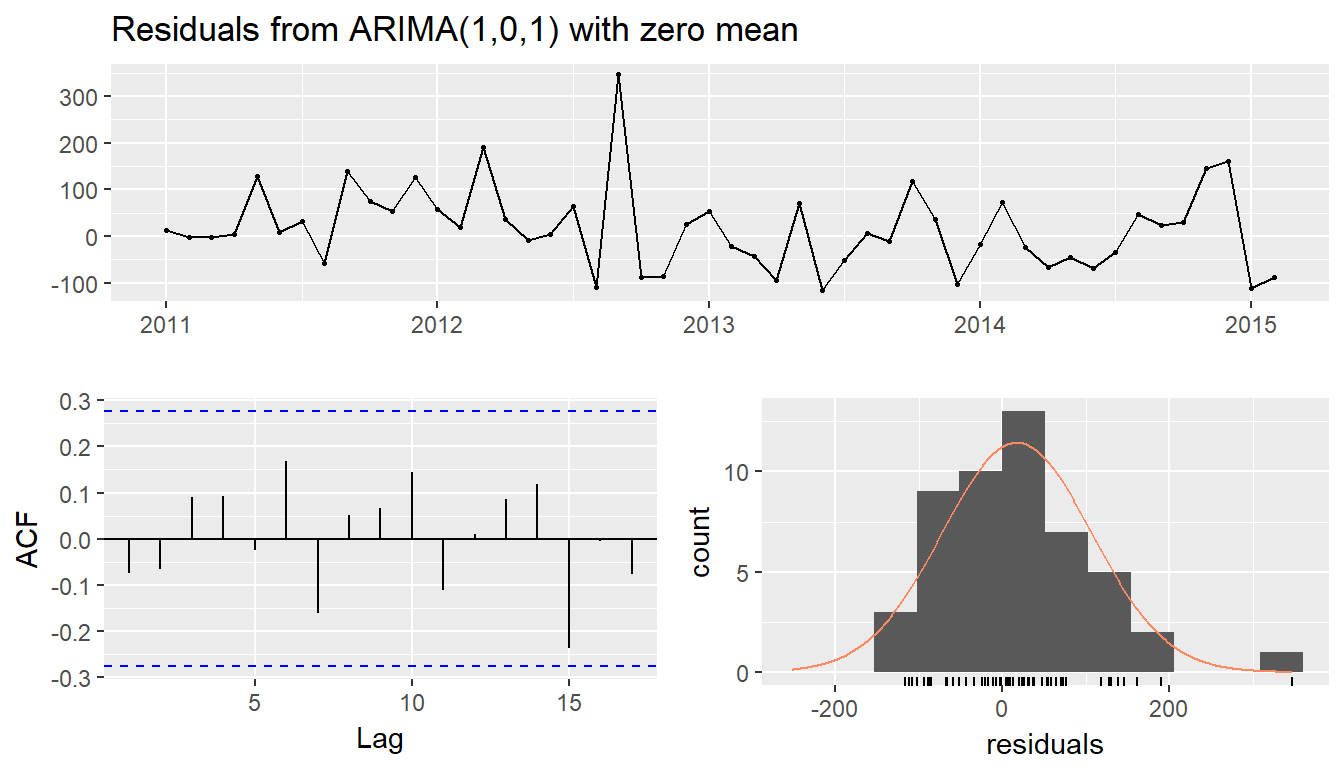Ljung-Box test

data:  Residuals from ARIMA(1,0,1) with zero mean
Q* = 19.786, df = 22, p-value = 0.5965

Model df: 2.   Total lags used: 24

Using the auto.Arima() function, I fitted an ARIMA model. This yields an ARIMA(1,0,1) model, which means that it is the combination of a first order autoregressive model ($$\phi$$ = 0.98) with no differencing and a first order moving average ($$\theta$$ = -0.57). This model seems reasonable given the sample ACF and PACF plots.

I considered adding a constant to the model, however that would lead to an overestimation of the long term trend of message volume. The zero mean assumption makes sense in that the limit of $$\widehat{y}_{t+h|t}$$ would approach a constant determined by the last few observations.

The autocorrelation seems appropriate, although the residuals do diverge visually from normal in QQ-plots. Nonetheless, given the amount of data we have I will accept this model.

### ETS Family

etsModel1 <- ets(train.ts)
checkresiduals(etsModel1)+theme_tufte()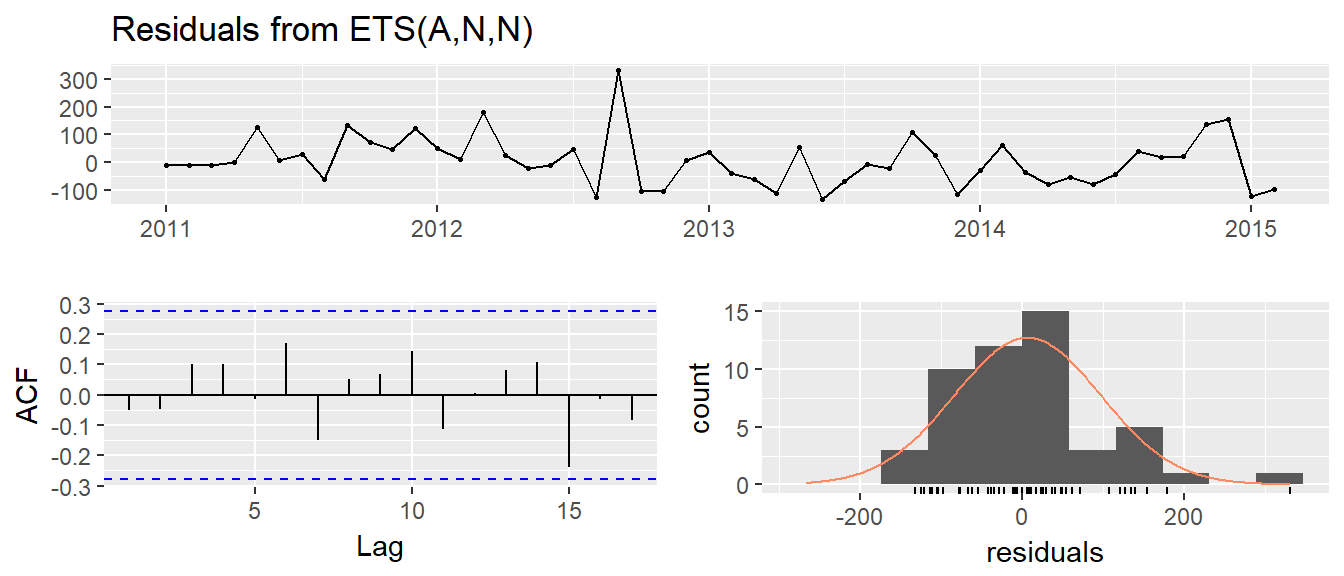##
Ljung-Box test
data:  Residuals from ETS(A,N,N)
Q* = 19.59, df = 22, p-value = 0.6087
Model df: 2.   Total lags used: 24

## NULL
fe1 <- forecast(etsModel1,h = 12)

The model selected is $$ETS(A,N,N)$$. The smoothing parameter is $$\alpha=$$ 0.42. This means that the growth, seasonal states do not change from their initial values. There is no damping parameter. Again, the residuals do not seem to be perfectly normally distributted, but this is not entirely surprising given the shortness of the time series. I will be content with the fact that they are not significantly non-normally distributed.

In order to select a model from these two I use time series cross validation following Hyndman’s example.

fets <- function(x, h) {
forecast(ets(x), h = h)
}
farima <- function(x, h) {
forecast(auto.arima(x), h=h)
}
e1 <- tsCV(train.ts, fets, h=1)#Compute CV errors for ETS as e1
e2 <- tsCV(train.ts, farima, h=1)#Compute CV errors for ARIMA as e2
Find MSE of each model class
mean(e1^2, na.rm=TRUE)

##  10710.59
mean(e2^2, na.rm=TRUE)
##  14473.21

Given that the MSE for the ETS model is lower, I would choose to select that to forecast.

## Model Evaluation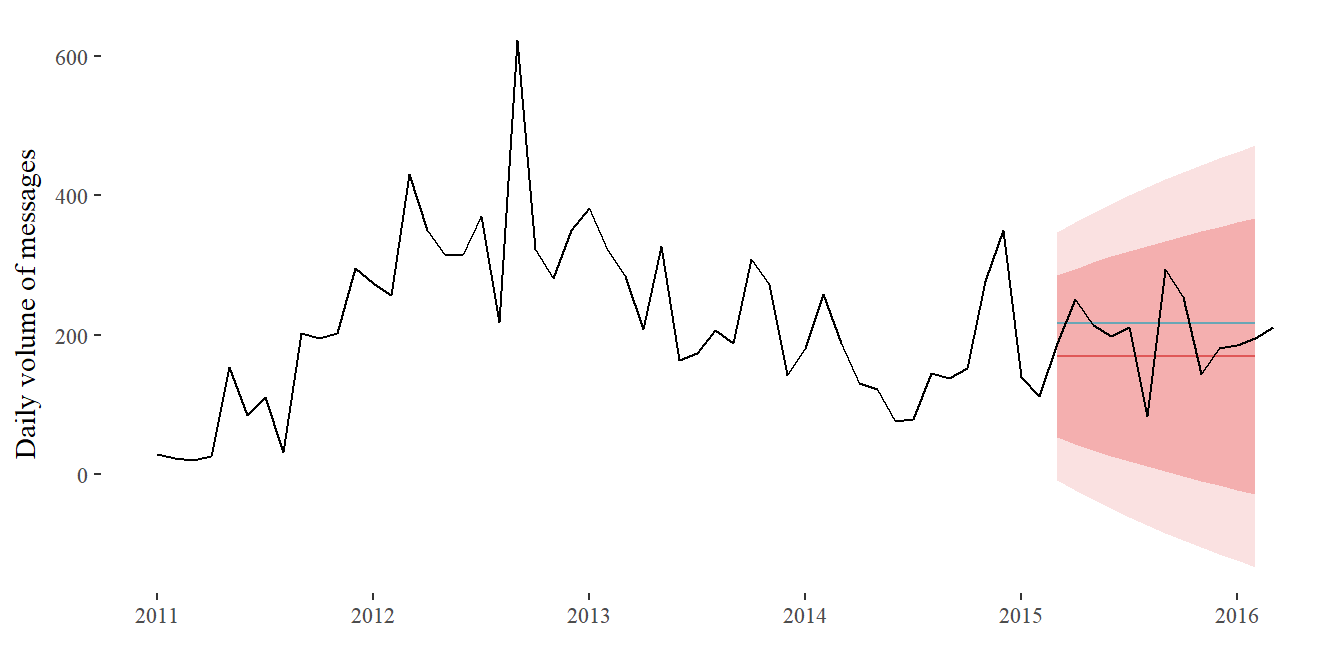In the plot above, forecasts compared to the test set. The ETS model estimates along with confidence intervals are presented in green, the mean forecast without confidence intervals is presented in blue.

Model evaluation for tested models
MERMSEMAEMPEMAPEMASEACF1Theil.s.UMethod
Training set1.71107.2273.41-10.9238.100.49-0.45NANaive
Test set87.17101.3691.9937.9043.630.61-0.270.97Naive
Training set10.00119.9196.93-86.07113.140.640.58NAMean
Test set1-16.2754.2241.29-18.9328.100.27-0.270.46Mean
Training set216.9190.4666.85-2.8935.410.44-0.07NAARIMA(1,0,1)
Test set257.4376.8967.3921.7733.610.45-0.310.79ARIMA(1,0,1)
Training set36.3290.3067.82-10.8137.650.45-0.05NAETS(A,N,N)
Test set330.6060.1049.226.8226.810.33-0.270.63ETS(A,N,N)

As expected, the ETS(A,N,N) model outperforms the ARIMA(1,0,1) model. However, using the test data we can see that none of the models perform particularly well. In fact, this seems to be one of those cases where the mean baseline model performs best in terms of RMSE.

## Packages Used

Arnold, Jeffrey B. 2017. Ggthemes: Extra Themes, Scales and Geoms for ’Ggplot2’. https://CRAN.R-project.org/package=ggthemes.

Aust, Frederik. 2016. Citr: ’RStudio’ Add-in to Insert Markdown Citations. https://CRAN.R-project.org/package=citr.

Francois, Romain. 2017. Bibtex: Bibtex Parser. https://CRAN.R-project.org/package=bibtex.

Grolemund, Garrett, and Hadley Wickham. 2011. “Dates and Times Made Easy with lubridate.” Journal of Statistical Software 40 (3): 1–25. http://www.jstatsoft.org/v40/i03/.

Hyndman, Rob J. 2017. forecast: Forecasting Functions for Time Series and Linear Models. http://pkg.robjhyndman.com/forecast.

Hyndman, Rob J, and Yeasmin Khandakar. 2008. “Automatic Time Series Forecasting: The Forecast Package for R.” Journal of Statistical Software 26 (3): 1–22. http://www.jstatsoft.org/article/view/v027i03.

R Core Team. 2017a. R: A Language and Environment for Statistical Computing. Vienna, Austria: R Foundation for Statistical Computing. https://www.R-project.org/.

———. 2017b. R: A Language and Environment for Statistical Computing. Vienna, Austria: R Foundation for Statistical Computing. https://www.R-project.org/.